# Solve the problem involving complex numbers

• chwala

#### chwala

Gold Member
Homework Statement
See attached.
Relevant Equations
Complex Numbers
Hello guys,
I am refreshing on complex numbers today; kindly see attached.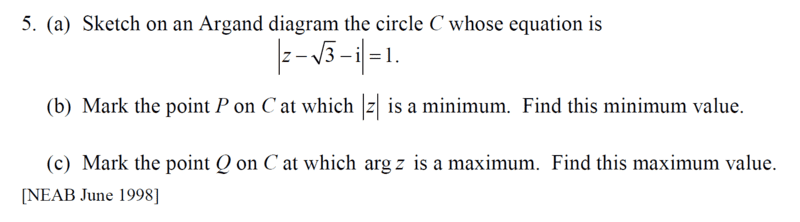ok for part (a) this is a circle with centre ##(\sqrt{3}, -1)## with radius =##1## thus, we shall have,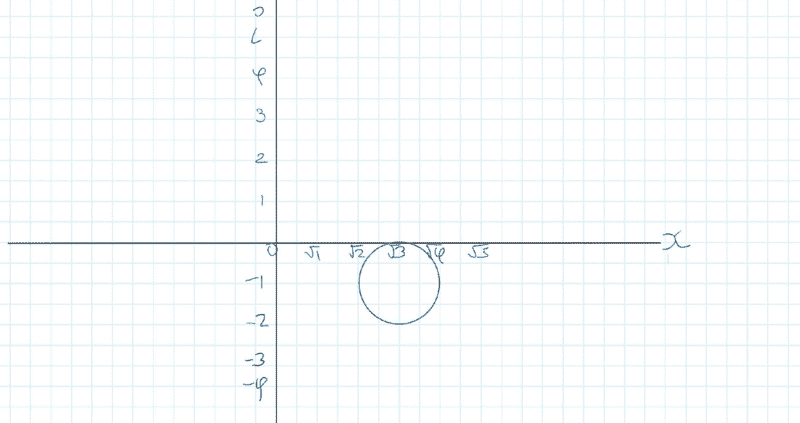Homework Statement:: See attached.
Relevant Equations:: Complex Numbers

Hello guys,
I am refreshing on complex numbers today; kindly see attached.

View attachment 324069

ok for part (a) this is a circle with centre ##(\sqrt{3}, -1)## with radius =##1## thus, we shall have,

View attachment 324070
Good!

Now, ##|z| = r## is, again, the circle in the Argand plane that has a radius r.

So, what is the radius of the smallest circle about the origin that intersects the circle you drew in part (a)?

Can you at least sketch this circle?

-Dan

•chwala
For part b,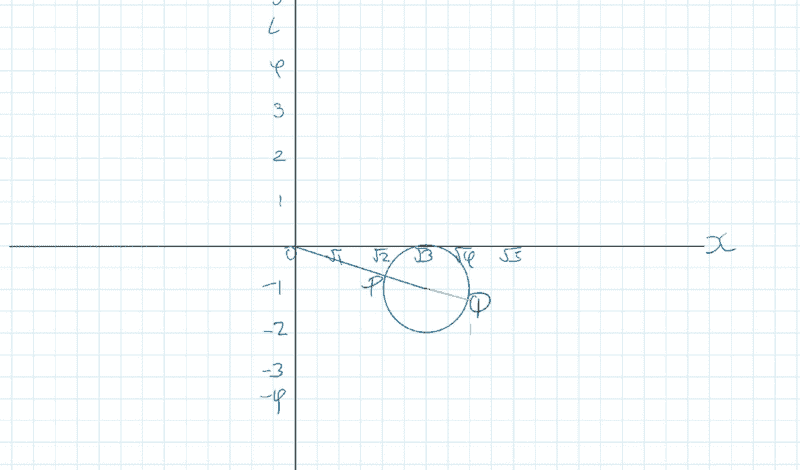The distance between the origin and the centre of the circle is ##|\sqrt {3}-i|=2## and the distance between point ##P## and centre of the circle is ##r=1##, therefore the Minimum value is ##2-1=1##

For part (c),

##\tan θ=\frac {-1}{\sqrt{3} }=-0.57735##

##θ=30^0##

therefore,

##θ_{Required}=330^0##

#### Attachments

ok for part (a) this is a circle with centre ##(\sqrt{3}, -1)## with radius =##1##
No, the center is at ##(\sqrt 3, i)##. ##z - \sqrt 3 - i = z - (\sqrt 3 + i)##.

•chwala
No, the center is at ##(\sqrt 3, i)##. ##z - \sqrt 3 - i = z - (\sqrt 3 + i)##.
@Mark44 ... I will check this again. Thanks mate.

...we shall now have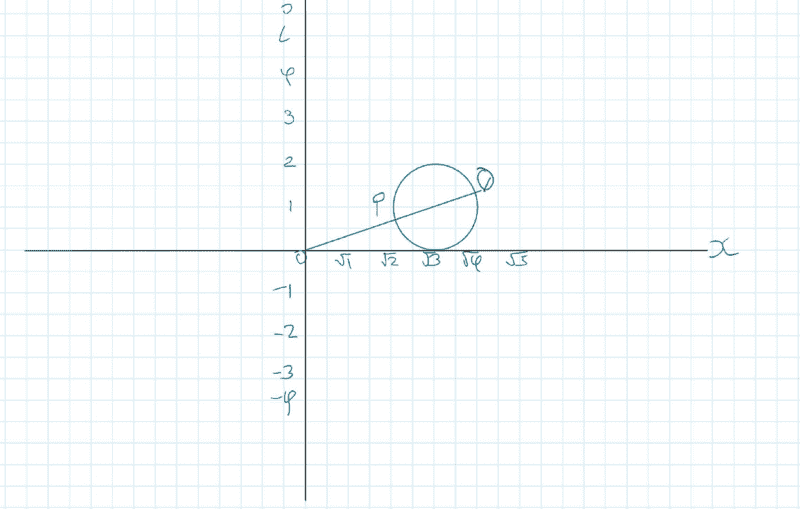For part (b)

The distance between the origin and the centre of the circle is ##|\sqrt {3}+i|=2## and the distance between point ##P## and centre of the circle is ##r=1##, therefore the Minimum value is ##2-1=1##

For part (c),

##\tan θ=\frac {1}{\sqrt{3} }=0.57735##

##θ=30^0##

For part (c),
##\tan θ=\frac {1}{\sqrt{3} }=0.57735##
##θ=30^0##
No, Q is the point on the circle at which arg z has its largest value. That is, the point Q at which the angle is largest. It's more than 30 °. Also, ##(\sqrt 3, 1)## is not a point on the circle.

Last edited:
No, Q is the point on the circle at which arg z has its largest value. That is, the point Q at which the angle is largest. It's more than 30 °. Also, ##(\sqrt 3, 1)## is not a point on the circle.

Yes, he's subtracting 1 from the center of the circle, and drawing a radial line from the center, which will hit the ( a point in the) circle.

@WWGD, I fixed your post where you had munged together what you wrote with the quote from me.

Yes, he's subtracting 1 from the center of the circle, and drawing a radial line from the center, which will hit the ( a point in the) circle.
But you probably realize that that doesn't give you the point on the circle where arg z is at its maximum.

But you probably realize that that doesn't give you the point on the circle where arg z is at its maximum.
No, I was referring to the shortest distance.

I think we might be talking about the same thing; namely, why arg z = 30° isn't what part c is asking for.

•chwala
For part (c),

tan⁡θ=13=0.57735
Then Θ would be the arg of P, through the center of the circle. Imagine a line from the origin O to Z, a point on the circle. Rotate that line CCW, increasing it's arg, when is it the greatest angle you can make with the x-axis and still be on the circle? What can you say about the geometrical relationship between that line OQ and the circle. Then what about the line CQ? Where O is the origin and C is the center of the circle. A little geometrical thinking can help here.

Also, it doesn't really matter, but... you've labeled the x axis with equal spacing between ##\sqrt{1}##, ##\sqrt{2}##, ##\sqrt{3}##, etc. That's not a linear plot and your circle would be distorted horizontally. My fix would be to erase all of them except ##\sqrt{3}##.

Last edited:
•chwala
No, Q is the point on the circle at which arg z has its largest value. That is, the point Q at which the angle is largest. It's more than 30 °. Also, ##(\sqrt 3, 1)## is not a point on the circle.
@Mark44 not getting you right... not a point on circle...my co ordinates are based on the complex plane...
For largest amplitude are we looking at ##210^0##? Thanks mate.

I think we might be talking about the same thing; namely, why arg z = 30° isn't what part c is asking for.
Ok now I seem to get you...let me look at this and get back ....

...Should be ##60^0##.

•DaveE
Note that you can solve your problems analytically by using the equation for a circle with radius 1 with centre at ##(\sqrt{3},1)##

##1^2 = (x-\sqrt{3})^2 + (y-1)^2##

Finding the point on the circle with min distance to the origin translates to solving for the minumum of ##x^2 + y^2## given ##1 = (x-\sqrt{3})^2 + (y-1)^2##.

And finding max argument translates to finding maxium ratio ##\dfrac{y}{x}## given ##1 = (x-\sqrt{3})^2 + (y-1)^2##.

•chwala
...we shall now have

View attachment 324090

For part (b)

The distance between the origin and the centre of the circle is ##|\sqrt {3}+i|=2## and the distance between point ##P## and centre of the circle is ##r=1##, therefore the Minimum value is ##2-1=1##

For part (c),

##\tan θ=\frac {1}{\sqrt{3} }=0.57735##

##θ=30^0##

The scale on the real axis is wrong; you can't have $\sqrt{n}$ equally spaced for integer $n$ and still have $|z - a| = b$ look like a circle of radius $b$ centered at $a$.

As others have noted, the maximum of $\arg(z)$ occurs when the tangent to the circle intersects the origin.

•SammyS, chwala and Steve4Physics
The scale on the real axis is wrong; you can't have $\sqrt{n}$ equally spaced for integer $n$ and still have $|z - a| = b$ look like a circle of radius $b$ centered at $a$.

As others have noted, the maximum of $\arg(z)$ occurs when the tangent to the circle intersects the origin.
True, actual diagram is here: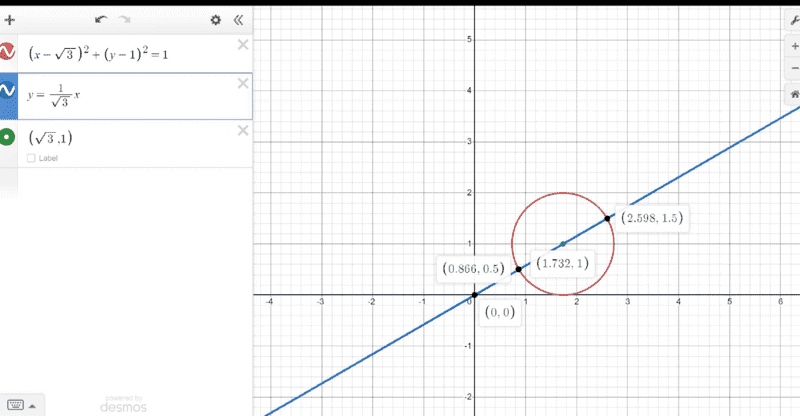#### Attachments

Note that you can solve your problems analytically by using the equation for a circle with radius 1 with centre at ##(\sqrt{3},1)##

##1^2 = (x-\sqrt{3})^2 + (y-1)^2##

Finding the point on the circle with min distance to the origin translates to solving for the minumum of ##x^2 + y^2## given ##1 = (x-\sqrt{3})^2 + (y-1)^2##.

And finding max argument translates to finding maxium ratio ##\dfrac{y}{x}## given ##1 = (x-\sqrt{3})^2 + (y-1)^2##.
I need time to look at how to solve this analytically. I know that i need to find the derivative of ##f(x,y)=0##. In essence, i need to substitute the given circle equation (preferably as one variable) into ##f(x,y)= x^2+y^2## , then differentiate...

I also tried to look at the Lagrange multiplier method ...interesting but the examples were more on straight line equations.

I think this is a bit straightforward...

copied from this site: kindly note the below is not my working.

https://www.quora.com/Is-it-possibl...enter-point-and-plane-of-the-circle-are-given

You asked for the max and min of three variables for a two dimensional object. Assuming the plane the circle is on is not titled in space only two variables will vary and have a max and min. If you know the radius and center coordinates of the circle, assuming the circle is in an x-y plane (with a constant z value), adding or subtracting the radius to the center coordinates will give the max and min values:

• center: (a, b)
• x-min: a - r
• x-max: a + r
• y-min: b - r
• y-max: b + r

And finding max argument translates to finding maxium ratio ##\dfrac{y}{x}## given ##1 = (x-\sqrt{3})^2 + (y-1)^2##.
Or equivalently, finding the maximum value of the derivative ##\frac{dy}{dx}##, where y is the y-coordinate on the circle in question. Note that if you solve the equation ##(x - \sqrt 3)^3 + (y - 1)^2 = 1## for y as a function of x, you get two values for most of the points on the circle, but the point on the circle where arg z is at its largest occurs on the upper half of the circle. The upper half can be written with y as a function of x.
You asked for the max and min of three variables for a two dimensional object. Assuming the plane the circle is on is not titled in space only two variables will vary and have a max and min. If you know the radius and center coordinates of the circle, assuming the circle is in an x-y plane (with a constant z value), adding or subtracting the radius to the center coordinates will give the max and min values:

• center: (a, b)
• x-min: a - r
• x-max: a + r
• y-min: b - r
• y-max: b + r
I don't see that any of this is relevant to finding the maximum value of arg z.

Last edited:
•malawi_glenn
I need time to look at how to solve this analytically. I know that i need to find the derivative of ##f(x,y)=0##. In essence, i need to substitute the given circle equation (preferably as one variable) into ##f(x,y)= x^2+y^2## , then differentiate...

I also tried to look at the Lagrange multiplier method ...interesting but the examples were more on straight line equations.
Just reduce it to a problem with one variable... solve for y and keep going

Or equivalently, finding the maximum value of the derivative ##\frac{dy}{dx}##, where y is the y-coordinate on the circle in question. Note that if you solve the equation ##(x - \sqrt 3)^3 + (y - 1)^2 = 1## for y as a function of x, you get two values for most of the points on the circle, but the point on the circle where arg z is at its largest occurs on the upper half of the circle. The upper half can be written with y as a function of x.

I don't see that any of this is relevant to finding the maximum value of arg z.
@Mark44 note that two tangents will intersect the circle and meet at the origin ##(0,0)## at an angle equal to ## 30^0 ×2=60^0##.

Note that we had already found the angle of ##30^0## using the trigonometry property of right angle as shown in my post ##6##.

I will amend my angles later into radians as advised by @pasmith ...later as I get hold of my computer.

Last edited by a moderator:
@Mark44 note that two tangents will intersect the circle and meet at the origin ##(0,0)## at an angle equal to ## 30^0 ×2=60^0##.
You needn't be concerned with the tangent on the lower half of the circle, as the angle there is less than the angle of the tangent at the upper left quadrant of the circle. Is the 60° the angle between the two tangents? If so, that's not what the problem is asking for -- it's the angle that the upper tangent makes with the horizontal axis.
chwala said:
Note that we had already found the angle of ##30^0## using the trigonometry property of right angle as shown in my post ##6##.
This isn't relevant. The angle you found is that angle made by a ray from the origin to the center of the circle.
chwala said:
I will amend my angles later into radians as advised by @pasmith ...later as I get hold of my computer.
I don't think it matters whether the angles are in radians or degrees -- more important is to realize which angle is being asked for.

You needn't be concerned with the tangent on the lower half of the circle
He's using that tangent to argue for similar (identical) triangles with the two tangents, their perpendiculars, and the central line. Alternatively you can construct the upper triangle, with the tangent you want and solve because you know the lengths of two sides, giving ##sin^{-1}(1/2)## for the upper triangle, then add that to the ##30^o## for the other one.

I have no idea how you got this. I'm pretty sure it's wrong, but I haven't worked this part of the problem.
????

He's using that tangent to argue for similar (identical) triangles with the two tangents, their perpendiculars, and the central line. Alternatively you can construct the upper triangle, with the tangent you want and solve because you know the lengths of two sides, giving ##sin^{-1}(1/2)## for the upper triangle, then add that to the ##30^o## for the other one.
You're right. What I was forgetting was that the circle was tangent to the x-axis. This makes the problem a lot simpler, one that doesn't require the use of derivatives.

@chwala, I agree now that max(arg z) is 60 degrees.

Last edited:
•chwala
@chwala :To nitpick a bit, maybe being somewhat pretentious, we should specify the choice of arg z . Is it Arg z, in ##(-\pi, \pi]##, or ##(\alpha, \alpha + \pi] ## for some ##\alpha \in \mathbb R##
Edit: And we can simplify by finding the distance between the center of the circle and the origin (or other point in question ) and subtract the radius, for the shortest distance.

Last edited:
•chwala
@chwala :To nitpick a bit, maybe being somewhat pretentious, we should specify the choice of arg z . Is it Arg z, in ##(-\pi, \pi]##, or ##(\alpha, \alpha + \pi] ## for some ##\alpha \in \mathbb R##
OK, true. But you may be the first person I ever heard say that [0, π/2] wouldn't be in the primary branch. It's hard to talk about Math if you have to include every possible restriction or contingency.

•Mark44
OK, true. But you may be the first person I ever heard say that [0, π/2] wouldn't be in the primary branch. It's hard to talk about Math if you have to include every possible restriction or contingency.
Fair-enough, but , for the sake of completeness, at least bring up the issue of the choice of arg. rigor without rigor mortis ;)

•PhDeezNutz